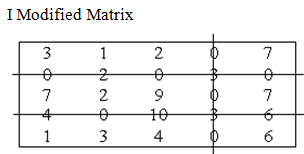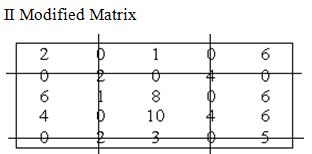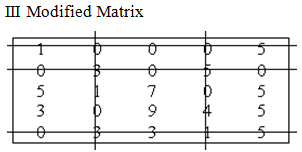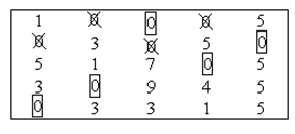#### Maximal Assignment Problem, Sample Assignments

Maximal Assignment Problem

Sample Assignment Problem

A company has 5 tasks to be performed. The following matrix presents the return in terms of rupees on allotting ith ( i = 1, 2, 3, 4, 5 ) machine to the jth job ( j = A, B, C, D, E ). Assign the five jobs to the five machines in order to maximize the total expected profit.

 Jobs Machines A B C D E 1 5 11 10 12 4 2 2 4 6 3 5 3 3 12 5 14 6 4 6 14 4 11 7 5 7 9 8 12 5

Subtract all the elements from the uppermost element

Highest element = 14

 9 3 4 2 10 12 10 8 11 9 11 2 9 0 8 8 0 10 3 7 7 5 6 2 9

Row Reduced matrix

 7 1 2 0 8 4 2 0 3 1 11 2 9 0 8 8 0 10 3 7 5 3 4 0 7

I Modified MatrixN < n i.e. 3 < 5

II Modified MatrixN < n i.e. 4 < 5

III Modified MatrixN = n

Zero assignmentOptimal assignment 1 - C   2 - E   3 - D   4 - B   5 - A

Maximum profit = 10 + 5 + 14 + 14 + 7 = Rs. 50